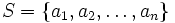# Two-line notation for permutations

## Definition

The two-line notation is a notation used to describe a permutation on a (usually finite) set.

### For a finite set

Suppose$S$ is a finite set and$\sigma:S \to S$ is a permutation. The two-line notation for$\sigma$ is a description of$\sigma$ in two aligned rows.

The top row lists the elements of$S$, and the bottom row lists, under each element of$S$, its image under$\sigma$.

If$S = \{ a_1, a_2, \dots, a_n \}$, the two-line notation for$\sigma$ is:$\begin{pmatrix}a_1 & a_2 & \dots & a_n \\ \sigma(a_1) & \sigma(a_2) & \dots & \sigma(a_n)\end{pmatrix}$.

The two-line notation for a permutation is not unique. Given a different enumeration for the set$S$, both rows change accordingly.

If the enumeration of the elements of$S$ is fixed once and for all, the top line can be dropped, giving rise to the one-line notation for permutations.

### For a countably infinite set

For a countably infinite set, we can use the two-line notation, with both lines being infinitely long.

## Examples

### Examples of the two-line notation for finite sets

Let$S =\{ 1,2,3,4 \}$ and$\sigma$ be defined as$\sigma(1) = 2$,$\sigma(2) = 4$,$\sigma(4) = 3$, and$\sigma(3) = 1$. The two-line notation for$\sigma$ is:$\begin{pmatrix}1 & 2 & 3 & 4 \\ 2 & 4 & 1 & 3 \\ \end{pmatrix}$.

### Examples of the two-line notation for infinite sets

Consider$S$ to be the set of all integers and$\sigma$ as the map$x \mapsto x + 1$. Then, the two-line notation for$\sigma$ is:$\begin{pmatrix}\dots & -2 & -1 & 0 & 1 & 2 & \dots \\ \dots & -1 & 0 & 1 & 2 & 3 & \dots \end{pmatrix}$.